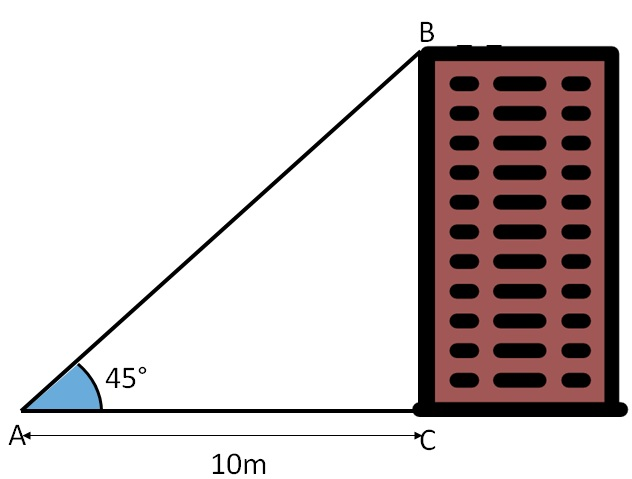Questions easy to difficult

Chapter 9 Class 10 Some Applications of Trigonometry
Concept wise
• Questions easy to difficult

Suppose angle of elevation from point A to the top of the tower is 45°. And distance from point A to the bottom of tower is 10m.

What is the height of the tower?

Let building be BCSo, ∠ BAC = 45°

and AC = 10 m

Now, we need to find height of tower i.e. BC

And we are given base 10 m.

So, we can use tan

As

tan A = Side opposite to angle A / Side adjacent to angle A

tan 45° = BC / AC

1 = BC/ 10

1 × 10 = BC

10 = BC

BC = 10 m

∴ Height of tower is 10 m

Get live Maths 1-on-1 Classs - Class 6 to 12